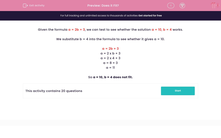# Does it Fit?

In this worksheet, students say whether or not the given solution fits the formula.Key stage:  KS 2

Curriculum topic:   Maths and Numerical Reasoning

Curriculum subtopic:   Equations and Algebra

Difficulty level:#### Worksheet Overview

Given the formula a = 2b + 3, we can test to see whether the solution a = 10, b = 4 works.

We substitute b = 4 into the formula to see whether it gives a = 10.

a = 2b + 3

a = 2 x b + 3

a = 2 x 4 + 3

a = 8 + 3

a = 11

So a = 10, b = 4 does not fit.

### What is EdPlace?

We're your National Curriculum aligned online education content provider helping each child succeed in English, maths and science from year 1 to GCSE. With an EdPlace account you’ll be able to track and measure progress, helping each child achieve their best. We build confidence and attainment by personalising each child’s learning at a level that suits them.

Get started••••